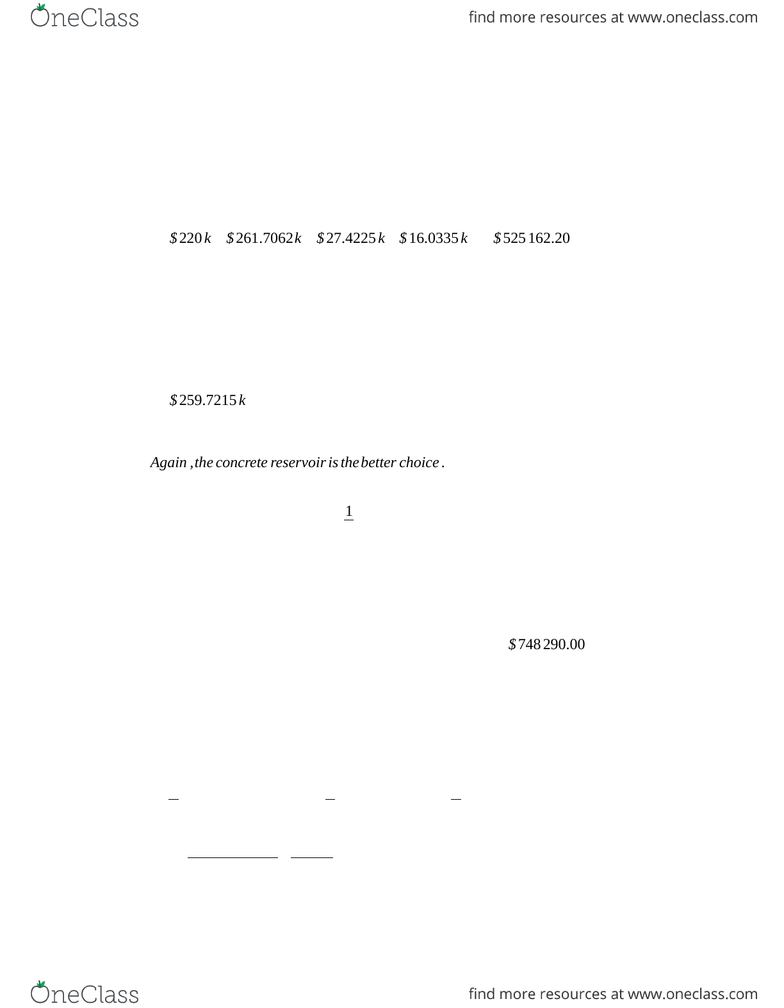Class Notes (1,100,000)
CA (620,000)
UTSG (50,000)
CHE (300)
Lecture 8

# CHE249H1 Lecture Notes - Lecture 8: Embankment Dam

Department
Chemical Engineering and Applied Chemistry
Course Code
CHE249H1
Professor
Yuri Lawryshyn
Lecture
8

Page:
of 21.
Present cost:
Concrete reservoir:
PW =\$300 k\$14 k ×
(
P/A ,5,20
)
=\$474 470.80
Earthen dam:
PW =\$220 k\$21 k ×
(
P/A , 5,20
)
\$35 k ×
(
P/F , 5,8
)
\$35 k ×(P/F ,5,16)
¿\$220 k\$261.7062k\$27.4225 k\$16.0335 k=\$525 162.20
Therefore, the concrete reservoir is less expensive and should be chosen.
Annual cost:
Concrete reservoir:
AW =\$300 k ×
(
A/P , 5,20
)
\$14 k=\$38 060.00 / yr
Earthen dam:
FC+Wood replacement=\$220 k\$35 k ×
(
P/F ,5,8
)
\$35 k ×
(
P/F ,5,16
)
¿\$259.7215 k
AW =\$259.7215 k ×
(
A/P , 5,20
)
\$21 k=\$41829.66 / yr
Again ,the concrete reservoir is thebetter choice .
2.
*Perpetual annuity
(
P/A , i , ∞
)
=1
i
Concrete reservoir:
Earthen dam:
Wood Replacement Annuity=−\$35 k ×
(
A/P ,5,8
)
=\$5 414.50 / yr
PW =\$220 k
[
\$21 k+\$5.41450 k
]
×
(
P/A ,5, ∞
)
¿\$748 290.00
3.
8.6 M, 4.3 M funded through bonds. F = 1000, n = 8 years, all semi-annual
Compare with:
P = 104, F = 100, CR = 5%, n = 6 years 3 months
Use this to /nd appropriate yield:
Start at 6 years, 6 months out, then move to 6 years 3 months
P=
(
F/P , λ
2,3months
)
[
\$2.5
(
P/A , λ
2,13
)
+\$100
(
P/F , λ
2,13
)
]
P=
(
1+λ
)
0.5
[
2.5
(
1+λ
)
n1
λ
(
1+λ
)
n+1001
(
1+λ
)
n
]
Try 4% (as P is slightly higher than F, implying CR is higher than yield)
This gives
λ
2=2
Using the actual formulas for everything, we get
λ=4.49
Then, we want 3300 bonds of \$1000 each to sell for at least \$4.3M, so each bond needs to sell for at
least \$1303.03
P=
[
\$ C
(
P/A , λ
2,13
)
+\$100 0
(
P/F , λ
2,13
)
]
Using goal seek, we see that we need a coupon value of \$49.58, which gets us to approximately 5%. This
would get us \$130.77 per \$100 face value.
The municipality would get
3300 bonds\$1307.72/bond=\$4,315 ,488.03
If there was a /nancing fee of 0.1%, we would need to raise 1.001*\$4.3M, which is \$4.3043 M, which is
less than the money our company would make anyways, so the price would not change. The municipality
however, would lose 0.1% of the bond sale to /nancing fees, so the municipality gets \$4,311,172.54.